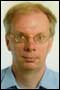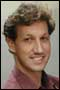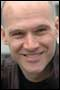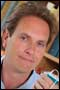Tjeerd M.H. Dijkstra, PhD, is a research scientist, Alexander Ypma, PhD, is senior research scientist, Bert de Vries, PhD, is principal scientist, and Jos R.G.M. Leenen, MScEE, is the director at GN ReSound’s Algorithm Research & Development Center in Eindhoven, The Netherlands.

This article discusses how hearing aid engineers have applied the Bayesian probability theory approach to the problem of hearing aid fitting. Currently more an art than a science, it is likely that probability theory will play a large role in future generations of fitting software used by dispensing professionals. We will show that probability theory is consistent with common-sense reasoning, a feature that is not shared by alternative mathematical frameworks for intelligent reasoning.

While probability theory gets to the same answers as a consistently reasoning human expert, it can deal with larger problems than a typical human is capable of handling. Since human expertise cannot be replaced by a mathematical system, we expect that mathematical reasoning systems, like the one described here, will serve as an assistant to the dispenser in difficult fitting tasks.

Who Was Bayes, and What is “Bayes’ Rule”?

Reverend Thomas Bayes (1702-1761) was a minister in Tunbridge Wells, England. Posthumously, his friend Richard Price published a paper titled “An Essay Towards Solving a Problem in the Doctrine of Chances” that contains a version of what is nowadays called “Bayes’ Rule.” For those readers who took statistics in college, most of you would not recognize this version as Bayes’ Rule; the credit commonly goes to the 1774 paper by the Frenchman Pierre-Simon Laplace (1749-1827).2

We will describe Bayes rule in the context of estimating a hearing instrument parameter setting. Assume that an algorithm parameter Θ can be set to any of K values, namely settings θ1, θ2, …, or θk. Therefore, we write:

P(Θ = θk)

…for the probability that qk is the preferred value for parameter Θ. For example, in the context of a noise reduction algorithm, P(Θ = θk) could denote the plausibility of three possible settings, a small (Θ = θ1), a medium (Θ = θ2), and a large amount (Θ = θ3) of noise reduction being preferred by a hearing aid user. Further, assume that, in general, half of the hearing aid users prefer the medium setting, and that the small and large settings are each preferred by one-quarter of the users. Making that assumption quantitative, we assign P(Θ = θ2) = 0.5 for the medium setting, and P(Θ = θ1) = P(Θ = θ3) = 0.25, for the small and large settings.

In determining the best setting for noise reduction, a clinician performs listening tests. The outcome of a listening test is quantified by:

P(D = dm | Θ = θk)

…which denotes the plausibility of outcome D = dm given that the user prefers Θ = θk (the vertical bar | is read as “given that”). For example, if we assume that the three possible responses are that the patient “likes” (D = d1), “doesn’t like” (D = d2), and “doesn’t know” (D = d3), then P(D = d1 | Θ = θ2) denotes the plausibility of response “like,” given that this user prefers the medium noise reduction setting.

What we would like to calculate is the plausibility that users prefer setting Θ = θk using the observed data from the listening tests. This plausibility is denoted by P(Θ = θk | D = dm) and is calculated by Bayes’ rule: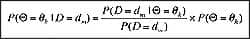The plausibility P(D = dm) is a normalization factor, and it is beyond the scope of this paper. But the important thing to recognize is that Bayes’ rule allows the calculation of plausibilities one wants to know (on the left side of the equation) from plausibilities that are known (on the right side).

Put another way, one learns a new plausibility from the data. More information on these principles can be found in the Wikipedia articles (www.wikipedia.org) on Bayes and Laplace.

The Bayesian Approach

The Bayesian approach has gained popularity in statistics, signal processing, and artificial intelligence. For instance, in the design and analysis of clinical trials, use of Bayesian statistics is becoming recognized by important entities like the FDA.1 In digital hearing aids, the algorithms employed in signal processing are starting to use techniques from Bayesian machine learning. Likewise, in modern fitting software, the use of artificial intelligence techniques is increasing. These areas of research are instrumental for improvements in hearing health care, and we expect dispensing professionals and consumers to benefit greatly from the Bayesian approach.

This paper explains the Bayesian approach, using a common problem in hearing aid fitting: how to set the gain in each frequency band. Starting from the scenario where a user returns to the dispenser with complaints about the hearing aid, we show how common-sense reasoning by a dispenser can illuminate the issues; subsequently, we show how it’s possible for a computer to reach the same conclusions.

One of the main points of this article is that this type of reasoning cannot be deductive, or based on classical logic; instead the reasoning used is inductive, based on probability theory—in particular Bayes’ rule (see sidebar, “Who Was Bayes and What Is Bayes’ Rule?”). State-of-the-art hearing aid algorithms that involve hundreds of adjustable parameters—which often influence the effects of other parameters—are not the best place to apply human problem-solving techniques. Reaching the appropriate parameter settings is not practical or even possible via common-sense human reasoning alone. Solutions can be found in Bayesian machine learning, which is designed to handle large quantities of uncertain data, using the same rules as common-sense human reasoning.

A Hearing Aid Fitting Problem

Shirley has just been fitted with a new hearing aid and has dinner that evening at a fine restaurant. She becomes somewhat disturbed by the clatter of her cutlery (knife and fork) on her plate. The sounds stand out more than what she is used to, and it annoys her. Later during the dinner, a live band moves around the restaurant. The low resonating sounds of the tuba make listening uncomfortable. The next day she visits the dispenser and tells her story. The dispenser is about to make some adaptations to her hearing aid parameter settings. What reasoning process will he follow to guide his decisions?

Human reasoning and probability theory. Before continuing, we should provide background on human reasoning, its relation to classical artificial intelligence (AI), and probability theory. In particular, it should be recognized that the rules of common-sense reasoning differ from deductive reasoning as used in classical AI.

What are the rules of logical reasoning? Since Aristotle (4th century BC), we have known that all true statements can be derived using only two rules:

We can read these rules as follows: The statement “If A is true, then B is true” is the premise, or the knowledge base. Suppose that a new fact becomes available, namely that A is indeed true. Then, according to Rule 1, it follows that B is true.

This kind of reasoning is called deductive reasoning and it forms the core of classical AI. Rule 2 says that, conversely, if we know that B is false (instead of knowing that A is true), it follows that A must also be false. No “ifs and buts” here. This is hard logic with binary outcomes: all statements are either true or false.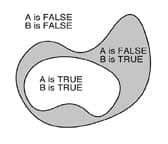FIGURE 1. Diagram of Statements A and B in an example of deductive logic.

More insight for these deductive rules can be gained from Figure 1. Since the area where B is true encompasses the area where A is true, it must be the case that B is true whenever A is true—essentially the same deduction as Rule 1. Insight into Rule 2 can be gleaned by noticing that the area where A is false encompasses the area where B is false.

We would love to use deductive reasoning for solving real-world problems. Unfortunately, many problems cannot be solved through deductive reasoning. We often have the premise “if A is true, then B is true,” combined with the observation “B is true” or “A is false.” For instance, in the Shirley example, we have the general rule “if the high-band gain is set too high, then high-frequency noise will sound too loud” combined with the observation “high-frequency noise sounds too loud.” Now what? There is no rule in deductive reasoning that we can use.

In this case, we need to reason backwards from observations to possible causes, which is a problem of induction. In inductive logic we add two more rules:

 Rules of Deductive Logic Rule 1 (Premise) if A is TRUE, then B is TRUE (Fact) A is TRUE (Conclusion) B is TRUE Rule 2 (Premise) if A is TRUE, then B is TRUE (Fact) B is FALSE (Conclusion) A is FALSE

 Extra Rules of Inductive Logic Rule 3 (Premise) if A is TRUE, then B is TRUE (Fact) B is TRUE (Conclusion) A becomes more plausible Rule 4 (Premise) If A is TRUE, then B is TRUE (Fact) A is FALSE (Conclusion) B becomes less plausible

These rules do not allow one to reach conclusions with certainty like the rules of deductive logic. Instead, these rules express a degree of plausibility through increasing or decreasing the plausibility of statements.

In daily life we often use these rules. For example, with the premise: if it rains, it is cloudy. Fact: it is cloudy in New York. Hence, it is more plausible that it rains in New York. In daily parlance, these rules are called common sense.

These statements can be made quantitative: we can assign a numerical value between 0 and 1 to the degree of plausibility of a statement. The truth-value “FALSE” corresponds to the number 0, and the truth-value “TRUE” corresponds to the number 1.

Assigning numerical values to the degree of plausibility raises the question as to how the degrees of plausibility should be combined. Cox3 has shown that probability theory is the only consistent mathematical system for combining all four rules of reasoning described above. This implies that any other mathematical system for doing intelligent reasoning either is consistent with probability theory (in which case it is redundant) or is inconsistent (in which case it can lead to strange answers).

Probability theory is an abstract mathematical formalism that defines the notion of “probabilities” and rules for combining them. In brief, probabilities are quantities between 0 and 1 that are combined with the product and sum rules (which are outside the scope of this paper). A mathematical probability P(A)—a number between 0 and 1—can be interpreted as the degree of plausibility that statement A is true.

The rules from probability theory for combining degrees of plausibility then form a calculus of plausible reasoning. Bayes’ rule is a direct consequence of probability theory, and hence represents a proven method for reasoning about degrees of plausibility. For a detailed discussion of these arguments, see Jaynes4 and the articles on “deductive reasoning” and “inductive reasoning” on Wikipedia.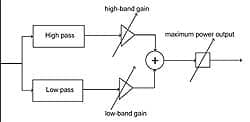FIGURE 2. Schematic of Shirley’s hypothetical hearing aid with only three adjustable parameters: high pass, low pass, and MPO.

Human reasoning about a hearing aid fitting problem. We will now explain why the reasoning process that our hypothetical dispensing professional follows cannot be based on deductive logic. He will use his common sense and show that this coincides with Rule 3, and hence with inductive reasoning. We again consider Shirley, who has just been fitted with a new hearing aid. For the sake of simplicity, we consider a hearing aid with just three tunable parameters (Figure 2), the gains in the low and high frequency bands, and the maximum power output level (MPO).

Remember, Shirley visited a restaurant and experienced two problems with her hearing aid. First, she noticed the annoyingly loud clatter of her knife and fork. Second, there was a live band playing in the restaurant, and she complained that the sound from the tuba was also uncomfortably loud. The dispenser reasons as follows: Shirley has trouble with both the high (knife clatter) and the low frequencies (tuba). He has three reasonable hypotheses that are consistent with these observations:

1. Both the high-band gain and the low-band gain are set too high, or
2. The MPO is set too high, or
3. All three are too high.

Let’s assume that the dispenser reasons that it is more plausible that one parameter (the MPO setting) is set to a wrong value rather than two parameters (both low-band and high-band gains) or all three parameters. This is a common-sense conclusion to a simple problem. Let us try to reason about this problem using deductive logic. As a first step, we assign labels to statements and start reasoning:

Possible cause HH = high-band gain is too high
Possible cause LH = low-band gain is too high
Possible cause MH = MPO is too high
Observation TL = tuba sounds too loud
Observation UL = cutlery noise too loud

The expert knowledge of the dispenser can be represented by a set of rules:

Knowledge Rule 1: If LH is TRUE, then TL is TRUE
Knowledge Rule 2: If MH is TRUE, then TL and UL are both TRUE
Knowledge Rule 3: If HH is TRUE, then UL is TRUEFIGURE 3. Bayes network for reasoning about Shirley’s hearing aid problems. Open circles denote variables that are not observed, and gray circles denote variables that are observed.

Figure 3 shows these statements and rules graphically in a so-called Bayes Network. The observations about the loud tuba and the clattering cutlery are interpreted by the dispenser as evidence for observations TL and UL.

Can he now deduct that LH (or HH or both LH and HH) is true? No, he cannot: given that both low and high frequency sounds are annoying, he cannot conclude anything about the gain settings using deductive logic.

The rules of deductive logic (Rules 1 and 2) do not apply here. Even in this simple problem, he must apply inductive logic. Since TL is observed, he concludes from inductive Rule 3 that LH (low-band gain too high) becomes more plausible. UL is also observed, hence he concludes that HH (high-band gain too high) is also more plausible. Since TL and UL are both observed, he concludes that it is also more plausible that the MPO was set too high.

So all possible causes are more plausible. But which one is most plausible? Intuitively, it seems that the explanation based on the level of the MPO is the most plausible, as it is supported by two observations. The other explanations are supported only by one observation and seem more coincidental.

As discussed, we need Bayes’ rule to draw quantitative conclusions about the relative plausibility of the possible causes. As calculated in the next section, Bayes’ rule shows that the most plausible cause is indeed that the MPO is set too high. (The sidebar “Three Kinds of Statistics” describes the relationship between Bayesian and conventional statistics, and how concepts from each can be used to build models for real-world problems.)

Bayesian Reasoning Applied to a Hearing Aid Fitting

In this section we provide a quantitative analysis of Shirley’s problem. Necessarily, this section is more technical than the previous ones. Thus, a reader who is content with the gist of the paper can skip to the next section. However, we provide a hands-on implementation of the Shirley example as a Bayes network using the freeware package GeNIe, allowing a reader to experiment with different choices of the parameters.

For full specification of the Bayes network as depicted in Figure 3, we need 11 numbers: three for the prior probabilities on each of the possible causes, and eight for the complaints (four for each complaint). The three priors denote how certain the dispenser is that he made the correct fit. The dispenser generally fits a hearing aid correctly. Thus, for the purpose of exposition, we set the probabilities for all causes to a small number, say 0.1. In formula, this can be represented as:

P(LH = TRUE) = 0.1
P(MH = TRUE) = 0.1
P(HH = TRUE) = 0.1

Next we specify the degree of plausibility that a complaint occurs for all possibilities of the causes. Focusing on the complaint that “the tuba sounds too loud,” we reason that, when both the low-band gain is too high and the MPO is too high, it is quite certain that the tuba will sound too loud, say 0.9. In formula:

P(TL = TRUE | LH = TRUE and MH = TRUE) = 0.9

Similarly, when just either of the possible causes is true, we assume it is a bit less likely that the tuba sounds too loud, say 0.8. In formula:

P(TL = TRUE | LH = TRUE and MH = FALSE) = 0.8
P(TL = TRUE | LH = FALSE and MH = TRUE) = 0.8

Lastly, when neither of the two possible causes is true, it is rather unlikely that the tuba sounds too loud, say 0.1. In formula:

P(TL = TRUE | LH = FALSE and MH =FALSE) = 0.1

We assume the network is symmetric, and thus the values are the same when the cutlery noise is too loud. In formula:

P(UL = TRUE | HH = TRUE and MH = TRUE) = 0.9
P(UL = TRUE | HH = TRUE and MH = FALSE) = 0.8>
P(UL = TRUE | HH = FALSE and MH = TRUE) = 0.8
P(UL = TRUE | HH = FALSE and MH =FALSE) = 0.1

We have now fully specified our knowledge base and are ready to reap the benefits.

Although it is relatively straightforward to calculate the plausibility of the possible causes by Bayes’ rule, it is more convenient to use the freeware package GeNIe (not to be confused with a proprietary hearing aid fitting software program). GeNIe can be downloaded for free from http://genie.sis.pitt.edu after a simple registration process.

The Shirley example as a Bayes network in the GeNIe environment closely resembles the graphic in Figure 3. Using GeNIe, we find a plausibility of 0.716 for the possible cause that the MPO is too high, and 0.213 for the alternative possibility that the low-band gain is too high. The possibility for the high-band gain being too high is also 0.213, since the network is symmetric by design. Thus, the level of the MPO as the source of the complaint is the most plausible cause, as expected.

Three Kinds of Statistics

Most people think there is just one kind of statistics—the kind you loved to hate in college. However, one can discriminate at least three different approaches to statistics in modern applications. Why are there different kinds of statistics at all? The answer is that statistics are about the relationships between the mathematical formalism of probability theory and the real world. Since one does not know the real world (only the measured data), one has to make assumptions about it. And different assumptions lead to different kinds of statistics.

Frequentist statistics has been the dominant type in the 20th century and is the kind we learned in college. It goes back to Neyman and Pearson who advocated its use in the 1930s. It is called “frequentist” since it interprets the outcome of an experiment as one of an infinite number of potential experiments. The p-value we constantly see in studies represents the frequency with which one can expect an outcome in the set of potential repeated experiments. Its advantages are its computational simplicity (an important bonus in the precomputer era) and its objectivity. Its disadvantage is the lack of flexibility and its peculiar interpretation: why does one need to imagine an infinite set of potential experiments just to interpret a single experiment?

Bayesian statistics was the dominant type in the 19th century, and has started to regain popularity in the 21st century. The advantages are highlighted in this article. One disadvantage is the computational burden, although this is hardly an issue with modern computing power. A second disadvantage could be that it is more work because one needs to specify priors (eg, “P(Θ = θk)” in the previous sidebar) for all relevant parameters. Alternatively, one could view this as an advantage, since it forces the modeler to be explicit about his or her assumptions. To confuse matters, even within the Bayesian community, there are several distinct viewpoints on how to interpret the concept of probability. In this paper, we adhere to the interpretation of probability as a state-of-knowledge. This is the original viewpoint of Bayes and Laplace, and was further developed by Jeffreys,5 Cox,3 and Jaynes.4

Machine learning is a modern branch of applied statistics and uses concepts from both frequentist and Bayesian statistics. Machine learning focuses on larger problems with practical applications in mind, and it mixes in methods from other fields like artificial intelligence and numerical analysis. Models built with Bayesian machine learning combine prior (expert) knowledge about a problem with measured data. The authors advocate the use of Bayesian machine learning as a framework for building consistent reasoning systems in hearing aids.

Given the relative complexity of the calculations, it seems natural to ask whether this overhead is necessary to reach such a straightforward conclusion. A first answer to this objection is that real problems have many more variables and cases, which limits the use of intuition. In contrast, Bayesian calculations can scale to far more complex problems with many variables.

A second answer is that there is an important payoff in the Bayesian approach: one can update the knowledge base with the knowledge that is acquired while diagnosing hearing aid fitting problems.

Learning of a Knowledge Base and Bayesian Prior Updating

In the previous section, we found that the Bayesian approach allows the dispenser to diagnose Shirley’s problem correctly (or, at least, according to human reason). One ingredient of the approach is the so-called “prior information” that constitutes the dispenser’s belief about the plausibility of incorrectly fitting a patient. The word “prior” refers to the notion that this is the dispenser’s initial belief before having diagnosed Shirley.

In the example, we set these priors to 0.1 for each of the possible causes. We would now like to update the dispenser’s prior beliefs, since the dispenser has found another incorrect fit: Shirley’s hearing aid needed adjustment. Obviously, the dispenser needs to increase his prior for the possible cause of setting the MPO too high. However, by how much should the dispenser increase his prior? Bayes comes to the rescue.

In fact, it is not so much Bayes as Laplace who came up with the answer, although it is based on Bayes’ rule (see first sidebar). We need one extra piece of information: the number of patients on which the dispenser’s prior is based. Say that the dispenser has seen 18 patients before Shirley and that one of them returned with problems similar to Shirley’s. According to Laplace’s rule of succession, the prior plausibility should be set to the number of misfits plus one (making a total of 2) divided by the total number of patients plus 2 (18+2 = 20—why the extra +1 and +2 are needed is outside the scope of this paper). Thus, the prior plausibility is 2/20 = 0.1 for the possible cause of MPO being too high.

As Shirley is the 19th patient seen by the dispenser, we can use Laplace’s rule of succession to update the prior. The number of misfits is now 2 and the number of patients is 19, leading to an updated prior of 3/21, which equals 0.143. Obviously, the number of previously seen patients influences how much the prior is updated by Shirley’s case. If the dispenser had seen 998 patients previously, 99 of whom had returned and needed an adjustment of the MPO, then the prior would be updated from 100/1,000 to 101/1,001, which is a small change.

One can view this updating of prior information as the learning of a knowledge base. The learned knowledge base contains information about the dispenser and his clients. As a consequence of the learning, if another person came along with identical complaints and an identical hearing aid, the fitting system would suggest that the MPO is too high with a plausibility larger than the 0.716 we calculated previously. Nothing in the mathematics of our example is specific to hearing instrument fitting and could just as well be applied in other areas (see the sidebar “Other Applications of Bayesian Statistics”).

We have exemplified the Bayesian approach with a scenario from a clinical practice of a dispenser, where a person returns with a complaint about the hearing instrument. We stress that the example in no way implies that the dispenser did anything incorrect. Fitting of hearing instruments is an art more than a science, and professional care will always be required. What the Bayesian approach brings is a bit more science to the art.

Other Applications of Bayesian Statistics

The Bayesian approach is gaining acceptance in clinical evaluations. In particular, the FDA has issued a guidance document1 allowing the use of Bayesian statistics in the clinical evaluation of medical devices, including hearing aids. The guidance document lists the following advantages of Bayesian statistics over frequentist statistics:

Sample size reduction or augmentation. The Bayesian methodology may reduce the sample size the FDA needs to reach a regulatory decision. You may achieve this reduction by using prior information and interim looks during the course of the trial. When results of a trial are unexpectedly good (or unexpectedly bad) at an interim look, you may be able to stop early and declare success (or failure). The Bayesian methodology can allow for augmentation of the sample in cases where more information helps the FDA make a decision. This can happen if the observed variability of the sample is higher than that used to plan the trial.

Midcourse changes to the trial design. With appropriate planning, the Bayesian approach can also offer the flexibility of midcourse changes to a trial. Some possibilities include dropping an unfavorable treatment arm or modifications to the randomization scheme. Modifications to the randomization scheme are particularly relevant for an ethically sensitive device (eg, animal studies) or when enrollment becomes problematic for a treatment arm. Bayesian methods can be especially flexible in allowing for changes in the treatment to control randomization ratio during the course of the trial.

Exact analysis. The Bayesian approach can sometimes be used to obtain an exact analysis when the corresponding frequentist analysis is only approximate or is too difficult to implement.

Other commercial applications. Other examples of a Bayesian approach can be found in diagnostics, like the Promedas system for medical diagnosis6 and the SKF BearingInspector system, a system for identifying bearing failures.7 Furthermore, Bayesian reasoning is at the basis of systems that have been used at NASA (eg, the decision-theoretic Vista system to support Mission Control),8 and several tools for automated data analysis and super-resolution imagery. Examples in the realm of computers are: Microsoft Lumière, the technology behind the user-requested services provided by the Office Assistant in MS Office, and Outlook Mobile Manager, a system that evaluates incoming e-mail messages and decides which ones are important enough to forward to a pager, mobile phone, or other e-mail address. Other applications that are often “powered by” Bayesian networks include speech recognition, robot vision, genetic linkage analysis, numerous tracking applications, and turbo-coding (third-generation mobile telephony protocols). Bayesian machine learning was listed as one of “10 emerging technologies that will change your world” in the February 2004 edition of MIT’s Technology Review.

User Preference Learning as Bayesian Prior Updating

As discussed in the introduction, the Bayesian approach could also be used in the signal processing of a hearing instrument. To illustrate this facet, we will discuss learning of the preference of the volume control: the learning volume control. Learning of a volume preference has been discussed before by Dillon et al,9,10 de Vries et al,11 Ypma et al,12 and Chalupper and Powers.13

To simplify things, we will consider a hypothetical learning volume control with only three levels +4 dB, 0 dB, and -4 dB. We also imagine a sound classifier inside the hearing instrument that classifies the incoming sounds into discrete categories: speech, music, and other. Many modern hearing instruments have a sound classifier in their signal processing. Since the classifier is not the focus of this paper, we simply assume that one exists. The question is how to associate a level of the learning volume control (say +4 dB) with a sound class (eg, music).

Things would be simple if users always preferred a certain volume level for a certain sound class. In that case, one could run a set of tests to establish user preference and be done. Unfortunately, sound classifiers make errors, users are not always consistent, and people may change their preferences over time. Consider the trainable hearing aid as proposed by Dillon et al,9,10 an instrument that uses responses from the user to adapt its settings. Dillon and colleagues propose this concept without specifying how the instrument can learn from user input. This section illustrates how the Bayesian approach could do that.

Adhering to the Bayesian approach, we associate a plausibility with each setting of the learning volume control. For example, P(LVC = +4 dB | sound = music) denotes the plausibility that the user prefers an extra 4 dB of amplification in the situation that the sound is classified as music. Since the mathematics is identical for each sound class, we assume the class is given and leave it out of the notation. Thus, for each sound class, we have three numbers—P(LVC = +4 dB), P(LVC = 0 dB), and P(LVC = -4 dB)—expressing the plausibility that the user prefers the associated level of amplification.

We imagine that the user can express preference for a certain level of the added gain by pressing a button repeatedly or by turning a volume control wheel. While the Bayesian approach also applies to a volume control wheel, the principles are more easily explained for a volume control with discrete levels. Further, imagine that the user presses the button once, expressing preference for the extra 4 dB of amplification. Clearly, we should increase the plausibility for P(LVC = +4 dB) and decrease the other two, since all three should sum to 1. However, by how much should P(LVC = +4 dB) be increased? By now, the answer should have a familiar ring to it: Bayes theorem tells us.

The problem is identical to the prior updating in the Shirley example. Thus, we need to keep track of how often the user preferred a certain level of the learning volume control, and use Laplace’s rule of succession: the plausibility is the number of button presses plus 1 divided by the total number of button presses plus 3. If this is the first button press by the user, there have been zero presses for the other two plausibilities and we get:

P(LVC = +4 dB) = (1 + 1) / (1 + 3) = 0.50
P(LVC = 0 dB) = (0 + 1) / (1 + 3) = 0.25
P(LVC = -4 dB) = (0 + 1) / (1 + 3) = 0.25

Which level of amplification should the user hear? The answer to this question is outside the scope of the Bayesian approach per se and is within the realm of decision theory. Two possibilities are the winner-take-all approach, where the user hears 4 dB of amplification, or the weighted average approach, where the user hears 0.5(+4) + 0.25(0) + 0.25(-4) = 1 dB.

The avid reader might have noticed that we gave two versions of Laplace’s rule of succession: in the previous example of updating the dispenser prior, we added +2 to the denominator, and here we add +3. Which is correct? The answer is that both are correct, as the number added to the total number of cases in the denominator should be the number of plausibilities considered (ie, +2 in the Shirley example, and +3 in this example).

The reader might have another objection to this formalism. Why use the Bayesian approach with Laplace’s rule of succession at all? It is much simpler to calculate the plausibility from the number of button presses for each level of the learning volume control divided by the total number of button presses:

P(LVC = +4 dB) = 1/1 = 1.0
P(LVC = 0 dB) = 0/1 = 0.0
P(LVC = -4 dB) = 0/1 = 0.0

Combined with the winner-take-all decision rule, there is no difference between this simple approach and the Bayesian approach. Using the weighted average decision rule, there is a difference, but is that all the advantage?

Bayes has one more twist. When sending a user off with a new hearing instrument, a dispenser would like to initialize the instrument to the best values. In the scenario above, it seems that best values are zero button presses for each of the three preferences. As a consequence, when the user has expressed his or her preference only a few times, large changes in the amplification are possible. One could say that this reflects the user’s preference and be done. Alternatively, one could obtain more information to bear on the matter of initialization.

Imagine that the hearing instrument manufacturer has run a field test with the instruments and found that users preferred the +4 dB amplification setting with a mean P(LVC = +4 dB) of 0.6 and a standard deviation of 0.2. How do we use this information in initializing the learning volume control?

Somehow, we have to convert the 0.6 and 0.2 (learned from the field trial) to a number of button presses. Since the user has not actually pressed the button yet, these are imagined button presses, indicating the most likely preference of the user. In Bayesian parlance, these button presses are called prior information. A calculation beyond the scope of this paper gives 3.6 prior button presses for the +4 dB preference and 1. for the other two.

Note that the button presses now are fractional numbers. Does that matter? Not really: these fractional prior button presses enter the calculation just as real button presses. What happens if a particular user prefers the 0 dB setting? Nothing other than that this user needs to indicate his preference for the 0 dB setting at least three times so that the prior for +4 dB (= 3.6) is overruled by the preference for 0 dB (1.2 + 3 = 4.2).

Summarizing, the Bayesian approach can be seen as a way to integrate information. Here we have seen how to integrate the information from one button press with information from previous presses and how to integrate information from a field trial with information obtained from an individual user.

Theory Becomes Application

Modern hearing aids have hundreds of parameters “under the hood.” A dispenser can no longer use only common sense and expert knowledge to cope with all the complexities and draw the optimal conclusions about parameter settings. GN ReSound is working on a Bayesian Fitting Assistant that suggests settings for the hearing aid algorithm parameters based on inputs, such as listening tests, auditory profile measurements, and expert knowledge.11,14 We envision this assistant to be an add-on to existing fitting software: a tool to aid dispensing professionals in optimizing the fitting and allow them to better counsel clients.

Using essentially the same mathematics, GN ReSound is also working on a Bayesian approach to integrate feedback information from the user to steer algorithms.12 We envision that users can provide rich information about their preferences to a future generation of hearing aids, either through a remote control during use or through listening tests in the dispenser’s office.

Conclusions

We have made a case for Bayesian probability theory as the foundation for solving hearing care problems in a quantitative matter. In 1812, Laplace wrote:

“The theory of probabilities is at bottom nothing but common sense reduced to calculus; it enables us to appreciate with exactness that which accurate minds feel with a sort of instinct for which oftentimes they are unable to account.”

—Laplace, 1812

Indeed, we have demonstrated—with the Shirley example—that Bayesian probability theory is consistent with common sense.

The fitting of a hearing aid with many tunable parameters is often too complex for common sense reasoning, but computers can carry out the calculations and assist the dispenser. An important benefit of Bayesian probability theory is that the system can learn from data by updating the prior knowledge base. We illustrated this in both the Shirley example (by learning an updated prior) and in the learning volume control example (by learning an updated user preference). In the latter case, we also showed how to integrate information from a field trial in the hearing aid.

In summary, we proposed plausibility from Bayesian probability theory as a currency to express knowledge and learning as the updating of these plausibilities. In the future, we anticipate that machine learning like this will produce a number of vital fitting assistants for dispensing professionals, both within the hearing instrument itself and within interface software for diagnostic, counseling, and fitting tools.

References

1. US Food and Drug Administration. Guidance for the Use of Bayesian Statistics in Medical Device Clinical Trials–Draft Guidance for Industry and FDA Staff, Docket number 2006D-0191. Washington, DC: FDA; 2006.
2. Laplace PS. Théorie analytique des probabilités. 1812.
3. Cox RT. Probability, frequency, and reasonable expectation. Am J Physics. 1946;14:1-13.
4. Jaynes ET. Probability Theory: The Logic of Science. Cambridge, UK: Cambridge University Press; 2003. Also available at: omega.albany.edu:8008/JaynesBook.html.
5. Jeffreys H. Theory of Probability. 3rd ed. Oxford, UK: Oxford University Press; 1998.
6. Kappen HJ, Wiegerinck WAJJ, Ter Braak E. Decision support for medical diagnosis. In: Meij J, ed. Dealing with the Data Flood. Mining Data, Text and Multimedia. The Hague: STT/Bewetong; 2002:111-121.
7. van der Vorst B, Schram G. Decision-support system for bearing failure mode. Evolution–SKF Bus Tech Mag. 2003;1/03:6.
8. Horvitz E, Barry M. Display of information for time-critical decision making. In: Proceedings of the 11th Conference on Uncertainty in Artificial Intelligence.1995:296-305.
9. Dillon K, Zakis JA, McDermott H, Keidser G, Dreschler W, Convery E. The trainable hearing aid: What will it do for clients and clinicians? Hear Jour. 2006;59(4):30-36.
10. Keidser G, Convery E, Dillon H. Potential users and perception of a self-adjustable and trainable hearing aid: A consumer survey. Hearing Review. 2007;14(4):18-31.
11. de Vries B, Ypma A, Dijkstra TMH, Heskes TM. Bayesian machine learning for personalization of hearing aid algorithms. Paper presented at: Intl Hearing Aid Research Conference; August 2006; Tahoe City, Calif.
12. Ypma A, de Vries B, Geurts J. Robust volume control personalization from on-line preference feedback. In: Proceedings of the IEEE Intl Workshop on Machine Learning for Signal Processing; September 2006; Maynooth, Ireland. 441-446.
13. Chalupper J, Powers TA. Changing how gain is selected: the benefits of combining datalogging and a learning VC. Hearing Review. 2006;13(13):46-55.
14. Heskes TM, de Vries B. In: Proceedings of Incremental Utility Elicitation for Adaptive Personalization, Belgian-Dutch Conference on Artificial Intelligence (BNAIC); October 2005; Brussels (Belgium):127-134.

Correspondence can be addressed to [email protected] or Tjeerd Dijkstra, GN ReSound A/S, Horsten 1, 5612 AX Eindhoven, The Netherlands; e-mail: .